# Fractions - 5th grade (10y) - math problems

#### Number of problems found: 51

• Ten fractionsWrite ten fractions between 1/3 and 2/3
• SchoolThere are 150 pupils in grade 5 . 2/3 of them are female. By what fractions are the males?
• Addition of mixed numeralsAdd two mixed fractions: 2 4/6 + 1 3/6
• FractionsSort fractions z1 = (6)/(11); z2 = (10)/(21); z3 = (19)/(22) by its size. Result write as three serial numbers 1,2,3.
• Cake fractions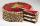Thomas ate 1/3 of cake, Bohouš of the rest of the cake ate 2/5. What fraction of cake left over for others?
• MilimetersHow many millimeters are 1/4 meters?
• Seeds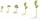Of the 72 wet seeds, 7/8 germinated. How many seeds germinate?
• Pounds3 pounds subtract 1/3 of a pound.
• Minutes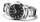Write as fraction in basic form which part of the week is 980 minutes.
• Women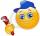In a company of 1050 employees are 2/3 women. How many women work in the company?
• Minute hand v2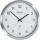In how many minutes describe the minute hand angle 60 degrees?
• Wage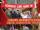Increasing the minimum wage while maintaining the other parameters of the system, unemployment will be:
• 3 children3 children had to divide 4 pounds is candy. How much candy did each child get?
• Cake 71/3 of a cake shared with 4 people. What share of the whole cake has each people?
• Jam cakes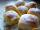Mom baked a third of plum jam cakes, one third cheesecakes and 18 poppy. How many cakes she had bake?
• Denominator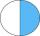Calculate the missing denominator x: (264)/(96) = (11)/(x)
• Roses and tulipsAt the florist are 50 tulips and 5 times fewer roses. How many flowers are in the flower shop?
• Cyclist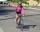Cyclist drives 5 km in half an hour, how long will drive in 3 hours?
• Cakes1/3 poppy cake, 1/3 apple, 15 pieces of cheese. How many are totally cakes?
• Icecreams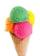Karthik bought 30 icecreams for his birthday .2/5 of them are chocolate icecreams. Find the number of chocolate icecreams he bought?

Do you have an interesting mathematical word problem that you can't solve it? Submit a math problem, and we can try to solve it.

We will send a solution to your e-mail address. Solved examples are also published here. Please enter the e-mail correctly and check whether you don't have a full mailbox.

Please do not submit problems from current active competitions such as Mathematical Olympiad, correspondence seminars etc...

Need help to calculate sum, simplify or multiply fractions? Try our fraction calculator. Fraction Word Problems. Examples for 5th grade (the fifth graders).